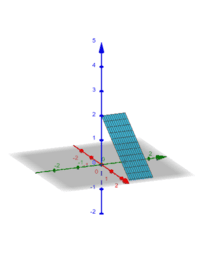Jila

•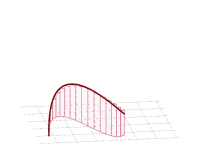Line Integral

Activity

Jila

•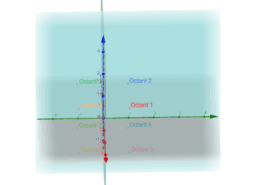Octants

Activity

Jila

•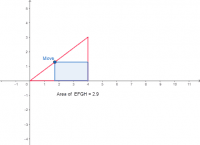4.7: Optimization: Inscribe a rectangle in a triangle

Activity

Jila

•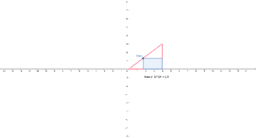3.2: Optimization: Inscribe a rectangle in a triangle

Activity

Jila

•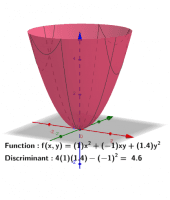Activity

Jila

•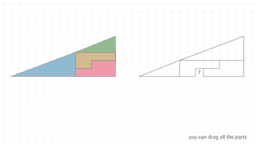2.1-Right triangle paradox, Created by Sonom

Activity

Jila

•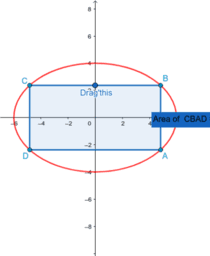4.7: Optimization- Maximize the area of a rectangle inscribed in an ellipse

Activity

Jila

•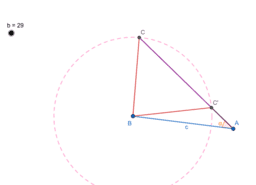8.1: SSA Sides a and b are given. Angle A is given. How many

Activity

Jila

•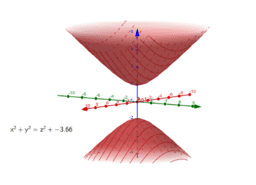Quadric Surfaces-Hyperboloids 1-Sheet, 2 -Sheets and Cones

Activity

Jila

•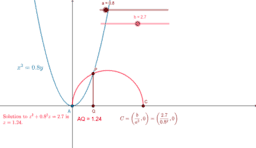Khayyam Equation Calculator

Activity

Jila

•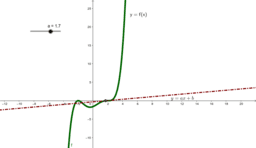Jila's Calculus class.

Book

Jila

•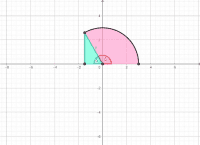WebAssign or Achieve Homework 15.4

Activity

Jila

•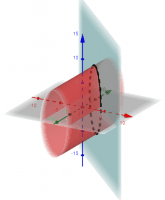Parametrization Examples

Activity

Jila

•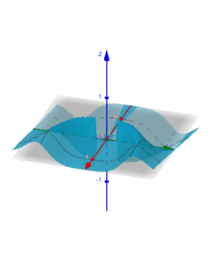xy^4/(x^2+y^8)

Activity

Jila

•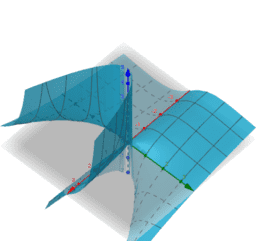xy/(x-y^2)

Activity

Jila

•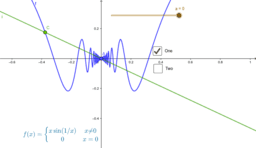3.1: Definition of Derivative for Oscillating Limits

Activity

Jila

•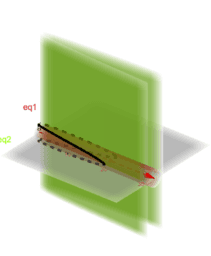Parametrization

Activity

Jila

•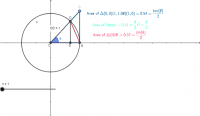2.6: Calculus 5.4: Precalculus How an angle and its sine are related (angles in first quadrant)

Activity

Jila

•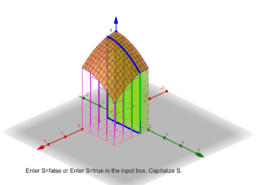Fubinni's 3d Riemann Sum

Activity

Jila

•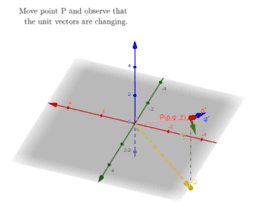The Unit Vectors in Physics, Spherical Coordinates

Activity

Jila

•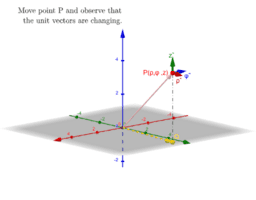The Unit Vectors in Physics, Cylindrical Coordinates

Activity

Jila

•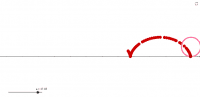8.7: Trace of a point on a wheel. Click play on the slider.

Activity

Jila

•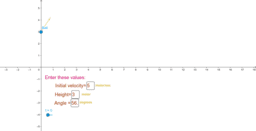Throwing a ball at an angle

Activity

Jila

•